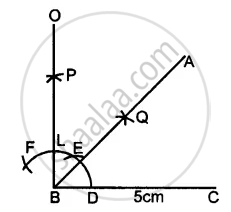# Construct Angle Abc = 45° in Which Bc = 5 Cm and Ab = 4.6 Cm. - Mathematics

Sum

Construct angle ABC = 45° in which BC = 5 cm and AB = 4.6 cm.

#### Solution

Steps of Construction :1. Draw a line segment BC = 5 cm
2. Taking B as centre, draw an arc of any suitable radius, which cuts BC at the point D.
3. With D as the centre and the same radius, as taken in step 2, draw an arc which cuts the previous arc at point E.
4. With E as the centre and the same radius, draw one more arc which cuts the first arc at point F.
5. With E and F as centres and radii equal to more than half the distance between E at F, draw an arc which cut each other at point P.
6. Join BP to meet EF at L and produce to point O. Then ∠OBC = 90°
7. Draw BA, the bisector of angle OBC. [With D, L as centres and suitable radius draw two arc meeting each other at Q produced it to R]
=> ∠ABC = 45° [∴ BA is bisector of ∠OBC ∴ ∠ABC = = 45°]
8. From BR cut arc AB = 4.6 cm
Concept: Construction of an Angle
Is there an error in this question or solution?
Chapter 18: Constructions - Exercise 18 (A) [Page 202]

#### APPEARS IN

Selina Concise Mathematics Class 8 ICSE
Chapter 18 Constructions
Exercise 18 (A) | Q 4 | Page 202
Share